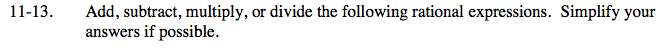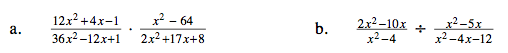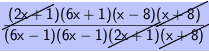### Home > CAAC > Chapter 11 > Lesson 11.1.1 > Problem11-13

11-13.Factor the expressions, then multiply them.

$\frac{(2\textit{x}+1)(6\textit{x}+1)}{(6\textit{x}-1)(6\textit{x}+1)}\cdot\frac{(\textit{x}-8)(\textit{x}+8)}{(2\textit{x}+1)(\textit{x}+8)}$

$\frac{(2\textit{x}+1)(6\textit{x}+1)(\textit{x}-8)(\textit{x}+8)}{(6\textit{x}-1)(2\textit{x}+1)(\textit{x}+8)}$

Look for factors that make 1.$\frac{(\textit{x}-8)}{(6\textit{x}-1)}$

Change division to multiplication, then see part (a).